# Get all rows in a Pandas DataFrame containing given substring

Let’s see how to get all rows in a Pandas DataFrame containing given substring with the help of different examples.

Code #1: Check the values PG in column Position

 `# importing pandas  ` `import` `pandas as pd ` ` `  `# Creating the dataframe with dict of lists ` `df ``=` `pd.DataFrame({``'Name'``: [``'Geeks'``, ``'Peter'``, ``'James'``, ``'Jack'``, ``'Lisa'``], ` `                   ``'Team'``: [``'Boston'``, ``'Boston'``, ``'Boston'``, ``'Chele'``, ``'Barse'``], ` `                   ``'Position'``: [``'PG'``, ``'PG'``, ``'UG'``, ``'PG'``, ``'UG'``], ` `                   ``'Number'``: [``3``, ``4``, ``7``, ``11``, ``5``], ` `                   ``'Age'``: [``33``, ``25``, ``34``, ``35``, ``28``], ` `                   ``'Height'``: [``'6-2'``, ``'6-4'``, ``'5-9'``, ``'6-1'``, ``'5-8'``], ` `                   ``'Weight'``: [``89``, ``79``, ``113``, ``78``, ``84``], ` `                   ``'College'``: [``'MIT'``, ``'MIT'``, ``'MIT'``, ``'Stanford'``, ``'Stanford'``], ` `                   ``'Salary'``: [``99999``, ``99994``, ``89999``, ``78889``, ``87779``]}, ` `                   ``index ``=``[``'ind1'``, ``'ind2'``, ``'ind3'``, ``'ind4'``, ``'ind5'``]) ` `print``(df, ``"\n"``) ` ` `  `print``(``"Check PG values in Position column:\n"``) ` `df1 ``=` `df[``'Position'``].``str``.contains(``"PG"``) ` `print``(df1) `

Output: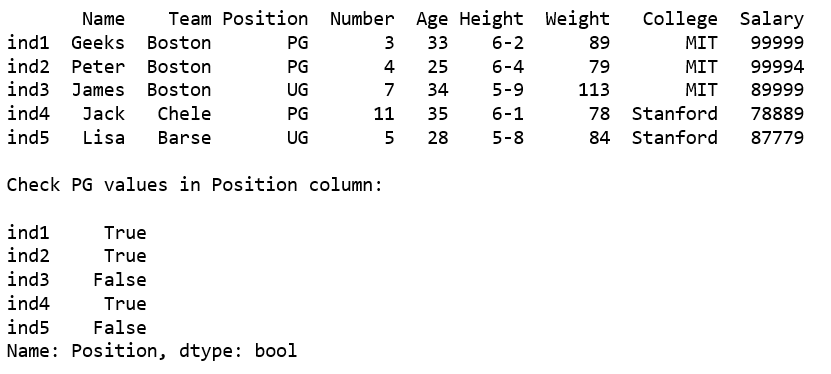But this result doesn’t seem very helpful, as it returns the bool values with the index. Let’s see if we can do something better.

Code #2: Getting the rows satisfying condition

 `# importing pandas as pd ` `import` `pandas as pd ` ` `  `# Creating the dataframe with dict of lists ` `df ``=` `pd.DataFrame({``'Name'``: [``'Geeks'``, ``'Peter'``, ``'James'``, ``'Jack'``, ``'Lisa'``], ` `                   ``'Team'``: [``'Boston'``, ``'Boston'``, ``'Boston'``, ``'Chele'``, ``'Barse'``], ` `                   ``'Position'``: [``'PG'``, ``'PG'``, ``'UG'``, ``'PG'``, ``'UG'``], ` `                   ``'Number'``: [``3``, ``4``, ``7``, ``11``, ``5``], ` `                   ``'Age'``: [``33``, ``25``, ``34``, ``35``, ``28``], ` `                   ``'Height'``: [``'6-2'``, ``'6-4'``, ``'5-9'``, ``'6-1'``, ``'5-8'``], ` `                   ``'Weight'``: [``89``, ``79``, ``113``, ``78``, ``84``], ` `                   ``'College'``: [``'MIT'``, ``'MIT'``, ``'MIT'``, ``'Stanford'``, ``'Stanford'``], ` `                   ``'Salary'``: [``99999``, ``99994``, ``89999``, ``78889``, ``87779``]}, ` `                   ``index ``=``[``'ind1'``, ``'ind2'``, ``'ind3'``, ``'ind4'``, ``'ind5'``]) ` ` `  `df1 ``=` `df[df[``'Position'``].``str``.contains(``"PG"``)] ` `print``(df1) `

Output: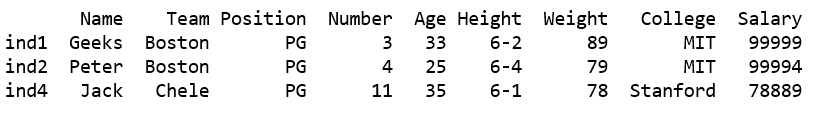Code #3: Filter all rows where either Team contains ‘Boston’ or College contains ‘MIT’.

 `# importing pandas ` `import` `pandas as pd ` ` `  `# Creating the dataframe with dict of lists ` `df ``=` `pd.DataFrame({``'Name'``: [``'Geeks'``, ``'Peter'``, ``'James'``, ``'Jack'``, ``'Lisa'``], ` `                   ``'Team'``: [``'Boston'``, ``'Boston'``, ``'Boston'``, ``'Chele'``, ``'Barse'``], ` `                   ``'Position'``: [``'PG'``, ``'PG'``, ``'UG'``, ``'PG'``, ``'UG'``], ` `                   ``'Number'``: [``3``, ``4``, ``7``, ``11``, ``5``], ` `                   ``'Age'``: [``33``, ``25``, ``34``, ``35``, ``28``], ` `                   ``'Height'``: [``'6-2'``, ``'6-4'``, ``'5-9'``, ``'6-1'``, ``'5-8'``], ` `                   ``'Weight'``: [``89``, ``79``, ``113``, ``78``, ``84``], ` `                   ``'College'``: [``'MIT'``, ``'MIT'``, ``'MIT'``, ``'Stanford'``, ``'Stanford'``], ` `                   ``'Salary'``: [``99999``, ``99994``, ``89999``, ``78889``, ``87779``]}, ` `                   ``index ``=``[``'ind1'``, ``'ind2'``, ``'ind3'``, ``'ind4'``, ``'ind5'``]) ` ` `  ` `  `df1 ``=` `df[df[``'Team'``].``str``.contains(``"Boston"``) | df[``'College'``].``str``.contains(``'MIT'``)] ` `print``(df1) `

Output: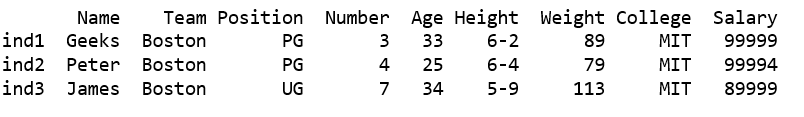Code #4: Filter rows checking Team name contains ‘Boston and Position must be PG.

 `# importing pandas module  ` `import` `pandas as pd  ` `   `  `# making data frame  ` `df ``=` `pd.read_csv(``"https://media.geeksforgeeks.org/wp-content/uploads/nba.csv"``)  ` ` `  ` `  `df1 ``=` `df[df[``'Team'``].``str``.contains(``'Boston'``) & df[``'Position'``].``str``.contains(``'PG'``)] ` `df1 `

Output: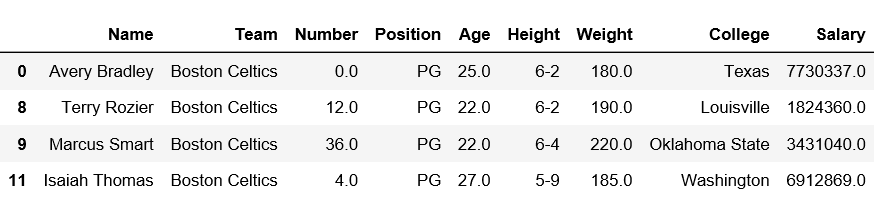Code #5: Filter rows checking Position contains PG and College must contains like UC.

 `# importing pandas module  ` `import` `pandas as pd  ` `   `  `# making data frame  ` `df ``=` `pd.read_csv(``"https://media.geeksforgeeks.org/wp-content/uploads/nba.csv"``)  ` ` `  ` `  `df1 ``=` `df[df[``'Position'``].``str``.contains(``"PG"``) & df[``'College'``].``str``.contains(``'UC'``)] ` `df1 `

Output: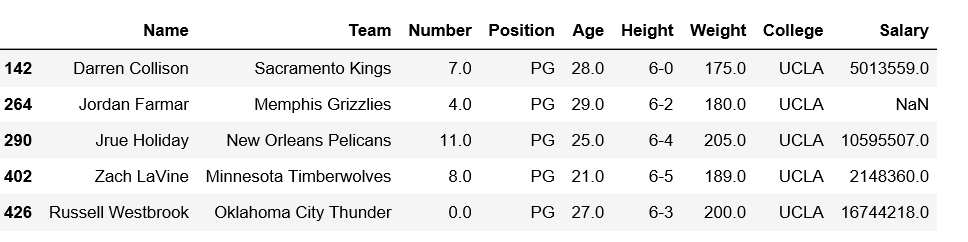My Personal Notes arrow_drop_upCheck out this Author's contributed articles.

If you like GeeksforGeeks and would like to contribute, you can also write an article using contribute.geeksforgeeks.org or mail your article to contribute@geeksforgeeks.org. See your article appearing on the GeeksforGeeks main page and help other Geeks.

Please Improve this article if you find anything incorrect by clicking on the "Improve Article" button below.

Article Tags :

2

Please write to us at contribute@geeksforgeeks.org to report any issue with the above content.